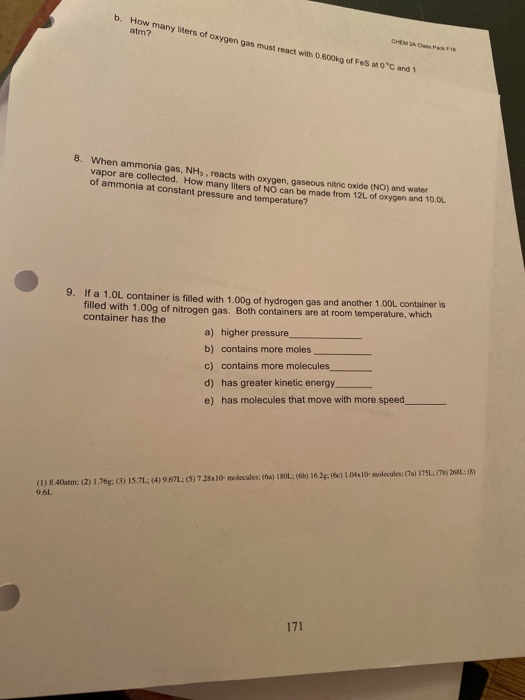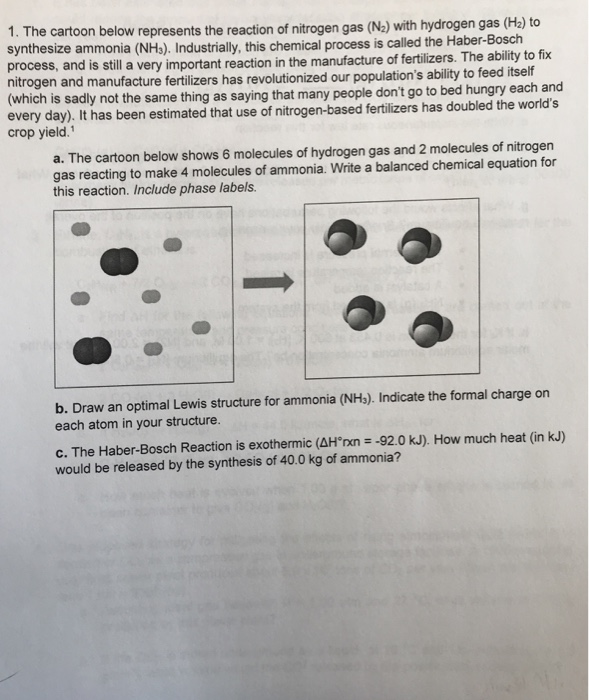# 1.Ammonia is created in the Haber process in a rigid container (nitrogen gas plus hydrogen gas react to form ammonia gas...

1.Ammonia is created in the Haber process in a rigid container (nitrogen gas plus hydrogen gas react to form ammonia gas). 2.0 moles of hydrogen gas are mixed with 4.0 moles of nitrogen gas. The initial pressure exerted on the container is 5.0 atm. Assuming the reaction runs to completion, what will the pressure (in atm) on the vessel be after the reaction takes place?

2.Pentane gas reacts with oxygen gas to give carbon dioxide gas and water vapor (gas). If you mix pentane and oxygen in the correct stoichiometric ratio, and if the total pressure of the mixture is 180 mm Hg, what are the partial pressures of pentane ( mmHg) and oxygen ( mm Hg)? If the temperature and volume do not change, what is the pressure of the water vapor ( mm Hg) after reaction

1.

The reaction between nitrogen gas and hydrogen gas to produce ammonia gas isIt is being assumed that the reaction runs to completion after starting with 2.0 moles of Hydrogen gas and 4.0 moles of nitrogen gas.

According to the balanced reaction, 1 mole of N2 gas reacts with 3 moles of H2 gas to form 2 moles of ammonia gas.

Hence, given thatThe pressure exerted is 5.0 atm initially when the number of moles of gas in the container isassuming constant volume and temperature.

Initial pressure in the container isHence, assuming the gases behave ideallyNote that V, T and R are all constants. Hence, the pressure in the container is only dependent on the number of moles of gas.

i.e pressure is directly proportional to the number of moles of gas. So we can writeThe number of moles of nitrogen gas that will react with 2.0 mol of H2 gas isHence, all 2.0 mol of H2 will react with 0.67 mol of N2 to form ammonia gas. Here H2 is the limiting reactant.

Note that 3 mol of H2 forms 2 mol NH3. Hence, the number of moles of ammonia gas formed from 2 mol H2 isHence, the constituents in the chamber after completion is(completely consumed as it is the limiting reactant)(nitrogen gas is in excess, hence, there is 3.33 mol leftover after completion)

Hence, the total number of moles of gas after completion of reaction is.

Hence, the final pressure in the container isHence, the pressure in the container at completion of reaction is about 3.9 atm.

2.

The balanced reaction between pentane and oxygen to produce water and CO2 isIt is given that pentane and oxygen are being mixed in correct stoichiometric ratio i.e. 1 mol of pentane per 8 mol of oxygen gas.

Hence, the mole fraction of pentane and oxygen in the mixture isTotal pressure of the pentane and oxygen gas mixture,Since the partial pressures of the gas is directly proportional to their mole fractions, the partial pressures are given byHence, the partial pressures of oxygen and pentane gas are 160 mmHg and 20 mmHg respectively.

After the reaction goes to completion at constant temperature and volume., we get 5 moles CO2 and 6 moles of H2O gases.(Note that since the reactant are taken in stoichiometric ratio, they are completely exhausted at completion)

We know that 9 moles of reactants resulted in a total pressure of 180 mmHg. Hence, 5 mol CO2 + 6 mol H2O =11 mol of products will result in a total pressure ofmole fraction of H2O gas at completion isHence, the partial pressure of H2O vapor at completion can be calculated asHence, the partial pressure of H2O at completion of reaction is 120 mmHg.

##### Add Answer of: 1.Ammonia is created in the Haber process in a rigid container (nitrogen gas plus hydrogen gas react to form ammonia gas...
Similar Homework Help Questions
• ### Nitrogen gas reacts with hydrogen gas to produce ammonia gas in a 25.0 L container. Initially,...

Nitrogen gas reacts with hydrogen gas to produce ammonia gas in a 25.0 L container. Initially, 0.5 molesof N2 and 3.0 moles of H2 are placed in the 25.0 L container, which is heated to 357 oC. What is the partialpressure of the ammonia gas product when the total gas pressure is 6.4 atm at 357 oC? Assume ideal gas behavior.a) 1.3 atmb) 1.7 atmc) 3.5 atmd) 0.61 atme) 0.83 atmi know the answer is e, but i dont know...

• ### In the Haber-Bosch process, nitrogen and hydrogen gas are reacted under high temperature 500 degrees C and pressure 200 atm to form ammonia gas (NH3) according to the following equation: N2+3H2 = 2NH3 If 9300 L H2 gas is reacted with an excess of N2 gas

In the Haber-Bosch process, nitrogen and hydrogen gas are reacted under high temperature 500 degrees C and pressure 200 atm to form ammonia gas (NH3) according to the following equation: N2+3H2 = 2NH3 If 9300 L H2 gas is reacted with an excess of N2 gas at 200 atm and 500 degrees C, how many moles of ammonia gas would be produced, assuming a 15% yield?

• ### Ammonia gas combines with oxygen to form nitrogen gas and water vapor

Ammonia gas combines with oxygen to form nitrogen gas and water vapor. If 234mL of ammonia are consumed in the reaction, how many liters of Nitrogen are produced?

• ### In the Haber process, ammonia is synthesized from nitrogen and hydrogen: N2 (g) + 3H2 (g) ? 2NH3...

In the Haber process, ammonia is synthesized from nitrogen and hydrogen:N2 (g) + 3H2 (g) ? 2NH3 (g)?G° at 298 K for this reaction is -33.3 kJ/mol. The value of ?G at 298 K for a reaction mixture that consists of 1.7 atm N2, 2.5 atm H2, and 0.55 atm NH3 is__________.

• ### 3.00 moles nitrogen gas and 3.00 moles oxygen gas react at 425 degrees celcius in a 30.5 L container to produce nitrogen dioxide

3.00 moles nitrogen gas and 3.00 moles oxygen gas react at 425 degrees celcius in a 30.5 L container to produce nitrogen dioxide. At the end of the chemical reaction, what will be the pressure in the container?

• ### b. How many liters of oxygen gas must react with 0.800kg of Fesmo" atm? CHEAP 01 8. When ammonia gas, NH, reacts wi...b. How many liters of oxygen gas must react with 0.800kg of Fesmo" atm? CHEAP 01 8. When ammonia gas, NH, reacts with oxyoen, gaseous nitric Oxide (NO) and water vapor are collected. How many liters of NO can be made from 12L of oxygen and 10.0 of ammonia at constant pressure and temperature? 9. If a 1.0L container is filled with 1.00g of hydrogen gas and another 1.00L container is filled with 1.00g of nitrogen gas. Both containers are...

• ### Ammonia gas is produced by reacting hydrogen gas with nitrogen gas...

Ammonia gas is produced by reacting hydrogen gas with nitrogen gas. To produce 856 g of ammonia gas using air as your source of nitrogen gas, what volume of air isrequired if the air is introduced at 27°C and 760 mmHg? (the mole fraction of nitrogen gas in air is 0.781)

• ### A sample of ammonia gas (NH3) is completely decomposed to nitrogen gas and hydrogen gas. If the...

A sample of ammonia gas (NH3) is completely decomposedto nitrogen gas and hydrogen gas. If the total pressure at the endof the reaction is 866mmHg, what isthe initial pressure of theammonia?HELP

• ### Ammonia gas is produced by reacting hydrogen gas with nitrogen gas. To produce 450 g of ammonia...

Ammonia gas is produced by reacting hydrogen gas with nitrogen gas. To produce 450 g of ammonia gas using air as your source of nitrogen gas, what volume of air isrequired if the air is introduced at 25°C and 740 mmHg? (the mole fraction of nitrogen gas in air is 0.781)A.534 LB.425 L C.290 LD.338 L

• ### 1. The cartoon below represents the reaction of nitrogen gas (N2) with hydrogen gas (H2) to synthesize ammonia (NHs). Industrially, this che pro mical process is called the Haber-Bosch cess...1. The cartoon below represents the reaction of nitrogen gas (N2) with hydrogen gas (H2) to synthesize ammonia (NHs). Industrially, this che pro mical process is called the Haber-Bosch cess, and is still a very important reaction in the manufacture of fertilizers. The ability to fix every day). It has been estimated that use of nitrogen-based fertilizers has doubled the world's a. The cartoon below shows 6 molecules of hydrogen gas and 2 molecules of nitrogen nitrogen and manufacture fertilizers...

Need Online Homework Help?